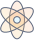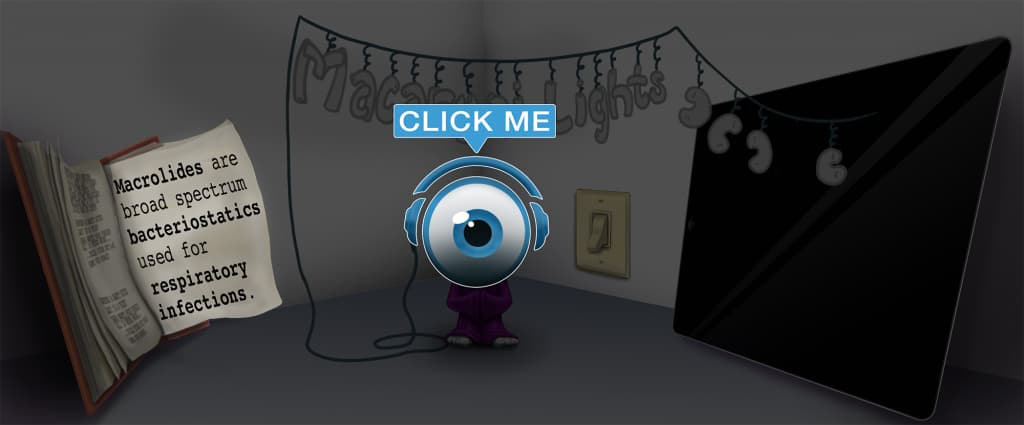# Master Parkland Formula with Picmonic for Medicine

## With Picmonic, facts become pictures. We've taken what the science shows - image mnemonics work - but we've boosted the effectiveness by building and associating memorable characters, interesting audio stories, and built-in quizzing.

### Parkland Formula

Park-LandPicmonic
The Parkland formula is used to calculate the amount of resuscitation fluid required in burn patients in the first 24 hours to ensure they remain hemodynamically stable. The amount of fluid in the first 24 hours is calculated by multiplying the total body surface area percentage burned (TBSA%) times the patient's mass in kg, times 4.
7 KEY FACTS
Burn victims
Burned victims

The Parkland Formula is used to calculate the amount of fluid given to burn victims.

24 hours
24 hour clock

This formula is used to calculate the amount of fluid given in the first 24 hours.

TBSA% X WEIGHT X 4 = FLUIDS
TBSA %
TBSA Agent

TBSA %, or total body surface area % burned, is approximated using the rule of 9's. This approximate percentage is then used in the Parkland formula.

x Weight (kg)
Kg Scale

The TBSA % is then multiplied against the patient's weight in kg.

x 4
(4) Fork

The patient's TBSA% x the patient weight in kg is then multiplied by 4.

= Fluid Requirement (in first 24 hrs)
Saline fluid

By multiplying the TBSA % x weight in kg x 4, we are able to calculate the burn victim's fluid requirement in the first 24 hours.

FLUIDS IN FIRST 8 HOURS
1/2 of fluid given in first 8 hours
Half of fluid drained from 8-bag

Of the total fluid which is calculated, the first ½ of the calculated fluid requirement is administered in the first 8 hours. The next ½ is then given over the period of 16 hours.

Unlock all 7 facts & definitions with Picmonic Free!

## Take the Parkland Formula Quiz

Picmonic's rapid review multiple-choice quiz allows you to assess your knowledge.

## Picmonic for Medicine CoversMedicine (MD/DO)
1,800+ Videos
21,000+ Facts

## Our Story Mnemonics Increase Mastery and Retention

Memorize facts with phonetic mnemonics

Unforgettable characters with concise but impactful videos (2-4 min each)

### Ace Your Medicine (MD/DO) Classes & Exams with Picmonic:

#### Over 1,240,000 students use Picmonic’s picture mnemonics to improve knowledge, retention, and exam performance.